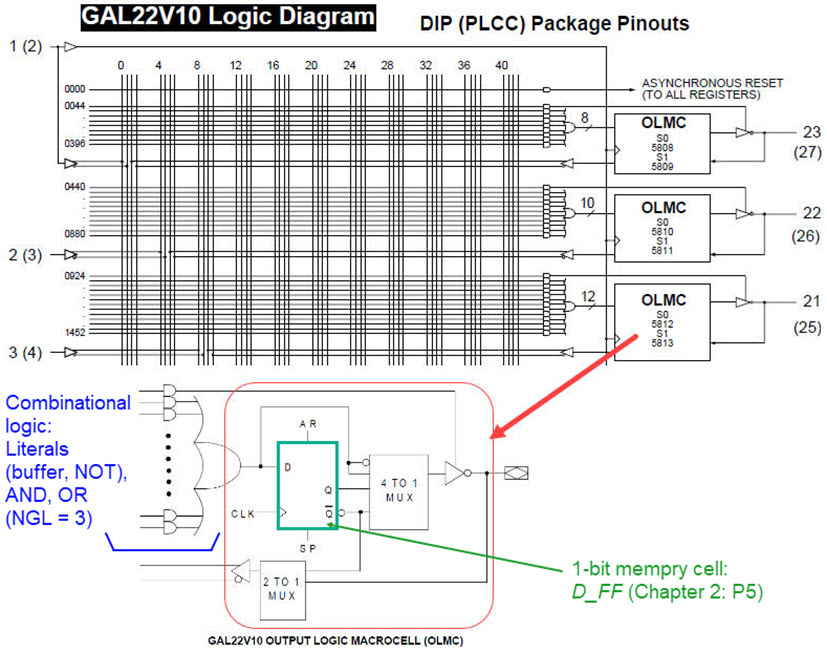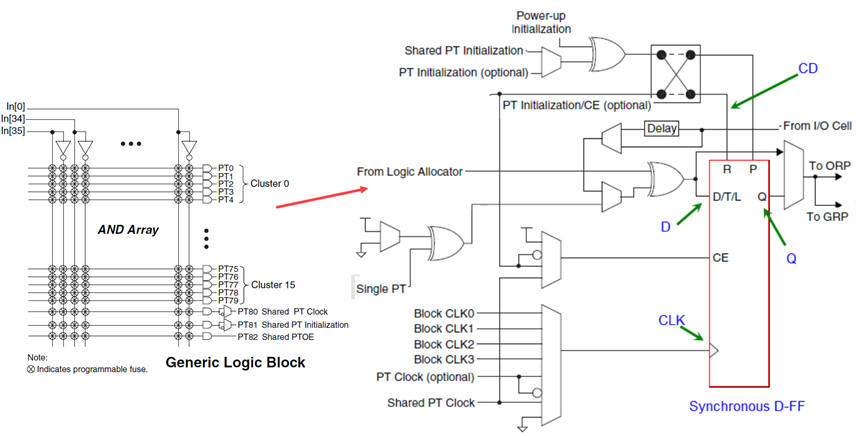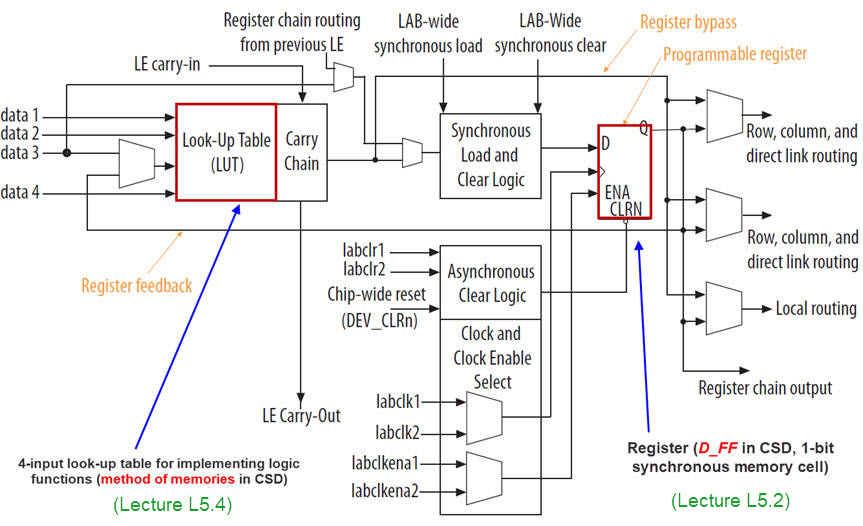﻿ Digital Circuits and Systems - Circuits i Sistemes Digitals (CSD) - EETAC - UPCBachelor's Degree in Telecommunications Systems and in Network Engineering## Programmable logic devices (synthesis target devices)

sPLD, CPLD and FPGA   (Chapters I and II)

Basic introduction to PLD and FPGA target chips. The wikipedia PLD entry.

### 1. sPLD: Simple PLD

The Wikipedia entry sPLD.Fig. 1. Example of logic array in sPLD.

### 2. CPLD: Complex PLD

The Wikipedia entry . Logic functions are implemented in PoS macrocells.Fig. 1. Example of CPLD logic cell.

### 3. FPGA

The Wikipedia entry FPGA. Logic functions are implemented using lookup tables (LUT), generally with a granularity of 4-input 1-bit RAM memory cells. How to implement logic functions using ?Fig. 1. FPGA logic element. Ref. Intel MAX10 10M50DAF484C7G.

Books

It is a good time for reading a book chapter on programmable logic devices. For instance, Chapter 11 in "INTRODUCTION TO LOGIC CIRCUITS & LOGIC DESIGN WITH VHDL", Brock J. LaMeres, 2nd edition, Springer, 2019, https://doi.org/10.1007/978-3-030-12489-2, available at the UPC library. Indeed, any on digital logic explains very well what is a programmable logic device able to implement combinational and sequential logic functions.

This is another recommended reading, specially the Chapter I on sPLD, CPLD and FPGA.